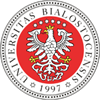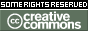REPOZYTORIUM UNIWERSYTETU
W BIAŁYMSTOKUProszę używać tego identyfikatora do cytowań lub wstaw link do tej pozycji: `http://hdl.handle.net/11320/6277`
 Tytuł: Basic Formal Properties of Triangular Norms and Conorms Autorzy: Grabowski, Adam Słowa kluczowe: fuzzy settriangular normtriangular conormfuzzy logic Data wydania: 2017 Data dodania: 8-lut-2018 Wydawca: DeGruyter Open Źródło: Formalized Mathematics, Volume 25, Issue 2, Pages 93–100 Abstrakt: SummaryIn the article we present in the Mizar system ,  the catalogue of triangular norms and conorms, used especially in the theory of fuzzy sets . The name triangular emphasizes the fact that in the framework of probabilistic metric spaces they generalize triangle inequality .After defining corresponding Mizar mode using four attributes, we introduced the following t-norms: minimum t-norm minnorm (Def. 6),product t-norm prodnorm (Def. 8),Łukasiewicz t-norm Lukasiewicz_norm (Def. 10),drastic t-norm drastic_norm (Def. 11),nilpotent minimum nilmin_norm (Def. 12),Hamacher product Hamacher_norm (Def. 13), and corresponding t-conorms: maximum t-conorm maxnorm (Def. 7),probabilistic sum probsum_conorm (Def. 9),bounded sum BoundedSum_conorm (Def. 19),drastic t-conorm drastic_conorm (Def. 14),nilpotent maximum nilmax_conorm (Def. 18),Hamacher t-conorm Hamacher_conorm (Def. 17). Their basic properties and duality are shown; we also proved the predicate of the ordering of norms , . It was proven formally that drastic-norm is the pointwise smallest t-norm and minnorm is the pointwise largest t-norm (maxnorm is the pointwise smallest t-conorm and drastic-conorm is the pointwise largest t-conorm). This work is a continuation of the development of fuzzy sets in Mizar  started in  and ; it could be used to give a variety of more general operations on fuzzy sets. Our formalization is much closer to the set theory used within the Mizar Mathematical Library than the development of rough sets , the approach which was chosen allows however for merging both theories , . Afiliacja: Institute of Informatics, University of Białystok, Poland URI: http://hdl.handle.net/11320/6277 DOI: 10.1515/forma-2017-0009 ISSN: 1426-2630 e-ISSN: 1898-9934 Typ Dokumentu: Article Występuje w kolekcji(ach): Artykuły naukowe (IInf)Formalized Mathematics, 2017, Volume 25, Issue 2

Pliki w tej pozycji:
Plik Opis RozmiarFormat
Pozycja ta dostępna jest na podstawie licencji Licencja Creative Commons CCL